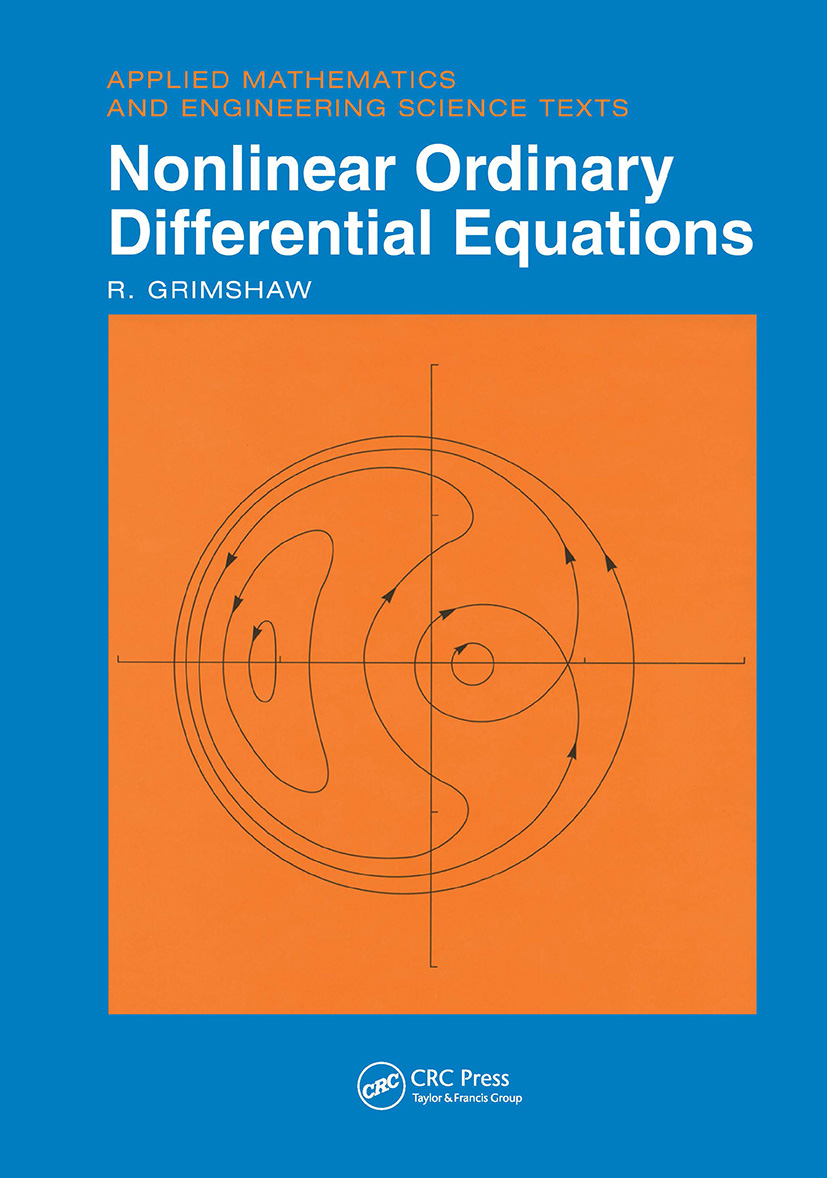Nonlinear Ordinary Differential Equations

1st Edition

CRC Press

336 pages

Purchasing Options:\$ = USD
Hardback: 9780849386077
pub: 1991-03-06
\$265.00
x
eBook (VitalSource) : 9780203745489
pub: 2017-10-19
from \$28.98

FREE Standard Shipping!

Description

Ordinary differential equations have long been an important area of study because of their wide application in physics, engineering, biology, chemistry, ecology, and economics. Based on a series of lectures given at the Universities of Melbourne and New South Wales in Australia, Nonlinear Ordinary Differential Equations takes the reader from basic elementary notions to the point where the exciting and fascinating developments in the theory of nonlinear differential equations can be understood and appreciated.

Each chapter is self-contained, and includes a selection of problems together with some detailed workings within the main text. Nonlinear Ordinary Differential Equations helps develop an understanding of the subtle and sometimes unexpected properties of nonlinear systems and simultaneously introduces practical analytical techniques to analyze nonlinear phenomena. This excellent book gives a structured, systematic, and rigorous development of the basic theory from elementary concepts to a point where readers can utilize ideas in nonlinear differential equations.

INTRODUCTION

Preliminary Notions

First-Order Systems

Uniqueness and Existence Theorems

Dependence on Parameters, and Continuation

LINEAR EQUATIONS

Uniqueness and Existence Theorem for a Linear System

Homogeneous Linear Systems

Inhomogeneous Linear Systems

Second-Order Linear Equations

Linear Equations with Constant Coefficients

LINEAR EQUATIONS WITH PERIODIC COEFFICIENTS

Floquet Theory

Parametric Resonance

Perturbation Methods for the Mathieu Equation

The Mathieu Equation with Damping

STABILITY

Preliminary Definitions

Stability for Linear Systems

Principle of Linearized Stability

Stability for Autonomous Systems

Liapunov Functions

PLANE AUTONOMOUS SYSTEMS

Critical Points

Linear Plane, Autonomous Systems

Nonlinear Perturbations of Plane, Autonomous Systems

PERIODIC SOLUTIONS OF PLANE AUTONOMOUS SYSTEMS

Preliminary Results

The Index of a Critical Point

Van der Pol Equation

Conservative Systems

PERTURBATION METHODS FOR PERIODIC SOLUTIONS

Poincaré-Lindstedt Method

Stability

PERTURBATION METHODS FOR FORCED OSCILLATIONS

Non-Resonant Case

Resonant Case

Resonant Oscillations for Duffing's Equation

Resonant Oscillations for Van der Pol's Equation

AVERAGING METHODS

Averaging Methods for Autonomous Equations

Averaging Methods for Forced Oscillations

Multi-Scale Methods

ELEMENTARY BIFURCATION THEORY

Preliminary Notions

One-Dimensional Bifurcations

Hopf Bifurcation

HAMILTONIAN SYSTEMS

Hamiltonian and Lagrangian Dynamics

Liouville's Theorem

Integral Invariants and Canonical Transformations

Action-Angle Variables

Action-Angle Variables: Perturbation Theory

ANSWERS TO SELECTED PROBLEMS

REFERENCES

INDEX.